# Predict Red Wine Quality with SVC, Decision Tree and Random Forest

## A Machine Learning Project with Python CodeRed Wine

# Dataset:

`df.info()`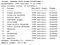Dataset Info
`df.head()`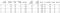First Five Rows

# Data Wrangling:

`df.isnull().sum()`
`fixed acidity           0 volatile acidity        0 citric acid             0 residual sugar          0 chlorides               0 free sulfur dioxide     0 total sulfur dioxide    0 density                 0 pH                      0 sulphates               0 alcohol                 0 quality                 0 dtype: int64`

# Data Exploration:

`import matplotlib.pyplot as pltimport seaborn as snsplt.figure(figsize=(30,20))corr = df.corr()sns.heatmap(corr,annot=True,cmap=sns.diverging_palette(200, 10, as_cmap=True))plt.show()`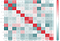Correlation Matrix
`plt.bar(df['quality'], df['alcohol'])plt.title('Relationship between alcohol and quality')plt.xlabel('quality')plt.ylabel('alcohol')plt.legend()plt.show()`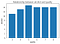Bar Graph

# Prepare the Data for Classification Model:

`from sklearn.preprocessing import MinMaxScalerscaler = MinMaxScaler(feature_range=(0, 1))normal_df = scaler.fit_transform(df)normal_df = pd.DataFrame(normal_df, columns = df.columns)print(normal_df.head())`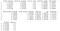Normalized Dataset
`df["good wine"] = ["yes" if i >= 7 else "no" for i in df['quality']]`
`X = normal_df.drop(["quality"], axis = 1)y = df["good wine"]`
`y.value_counts()`
`no     1382 yes     217 Name: good wine, dtype: int64`
`sns.countplot(y)plt.show()`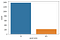Count plot

# Modeling:

`from sklearn.model_selection import train_test_splitX_train, X_test, y_train, y_test = train_test_split(X, y, test_size=0.3, random_state=2020, stratify=y)`

# Baseline Classification:

`from sklearn.dummy import DummyClassifierdummy_classifier = DummyClassifier(strategy='most_frequent',random_state=2020)dummy_classifier.fit(X_train,y_train)acc_baseline = dummy_classifier.score(X_test,y_test)print("Baseline Accuracy = ", acc_baseline)`
`Baseline Accuracy =  0.8645833333333334`

# Model One: Support Vector Classifier

## 1. Fit, Predict, and Accuracy Score:

`from sklearn.svm import SVCsvc = SVC(random_state=2020)svc.fit(X_train, y_train)`
`from sklearn import metricsfrom sklearn.metrics import accuracy_scorey_pred = svc.predict(X_test)print(metrics.accuracy_score(y_test, y_pred))`
`SVM Accuracy =  0.8854166666666666`

## 2. Overfitting:

Cross Validation (CV) estimates the generalized performance using the same data as is used to train the model. The idea behind cross-validation is to split the dataset up into a certain number of subsets, and then use each of these subsets as held out test sets in turn while using the rest of the data to train the model. (Source: Stack Exchange)

`from sklearn.model_selection import cross_val_scorescores = cross_val_score(svc, X, y, cv=5)print(scores.mean())`
`Cross Validation Score:  0.8642927115987462`
`y_pred_train = svc.predict(X_train)print(metrics.accuracy_score(y_train, y_pred_train))`
`Training Accuracy:  0.8927613941018767`

## 3. Tune the Model’s Parameters:

`from sklearn.model_selection import RandomizedSearchCVrandom_grid = {"C": [0.001,0.01,0.1,1,10,100,1000]}svc_random = RandomizedSearchCV(svc,random_grid,cv=5,random_state=2020)svc_random.fit(X_train, y_train)print(svc_random.best_params_)`
`{'C': 1}`
`from sklearn.model_selection import GridSearchCVparam_dist = {'C': [0.8,0.9,1,1.1,1.2,1.3,1.4],              'kernel':['linear', 'rbf','poly']}svc_cv = GridSearchCV(svc, param_dist, cv=10)svc_cv.fit(X_train,y_train)print(svc_cv.best_params_)`
`{'C': 1.3, 'kernel': 'rbf'}`
`svc_new = SVC(C = 1.3, kernel = "rbf", random_state = 2020)svc_new.fit(X_train, y_train)y_pred_new = svc_new.predict(X_test)print(metrics.accuracy_score(y_test, y_pred_new))`
`New SVM accuracy =  0.89375`

# Model Two: Decision Tree

## 1. Fit, Predict, and Accuracy Score:

`from sklearn.tree import DecisionTreeClassifierdt = DecisionTreeClassifier(random_state=2020)dt.fit(X_train, y_train)`
`from sklearn.metrics import plot_confusion_matrixy_pred = dt.predict(X_test)metrics.plot_confusion_matrix(dt, X_test, y_test)plt.show()print(metrics.accuracy_score(y_test, y_pred))`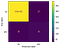Confusion Matrix
`Decision Tree Accuracy =  0.88125`

## 2. Overfitting:

`from sklearn import treeplt.figure(figsize=(40,20))fn = X.columnscn = y.unique()tree.plot_tree(dt, feature_names=fn, class_names=cn, filled=True)plt.show()`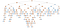Decision Tree
`scores = cross_val_score(dt, X, y, cv=5)print("Cross Validation Score: ",scores.mean())`
`Cross Validation Score:  0.8054917711598746`
`y_pred_train = dt.predict(X_train)print(metrics.accuracy_score(y_train, y_pred_train))`
`Training Accuracy: 1.0`

## 3. Tune the Model’s Parameters:

`param_dist = {"max_depth": range(1,6),              "max_features": range(1,10),              "criterion": ["gini", "entropy"]}dt_cv = GridSearchCV(dt, param_dist, cv=5)dt_cv.fit(X_train,y_train)print(dt_cv.best_params_)`
`{'criterion': 'gini', 'max_depth': 2, 'max_features': 8}`
`dt_new = DecisionTreeClassifier(criterion = "gini",                                max_depth = 2,                                max_features = 8,                                random_state = 2020)dt_new.fit(X_train, y_train)y_pred_new = dt_new.predict(X_test)print(metrics.accuracy_score(y_test, y_pred_new))scores = cross_val_score(dt_new, X, y, cv=5)print("Cross Validation Score: ",scores.mean())`
`New Decision Tree Accuracy:  0.8854166666666666New Cross Validation Score:  0.8786794670846394`
`plt.figure(figsize=(40,20))tree.plot_tree(dt_new, feature_names=fn, class_names=cn, filled=True)plt.show()`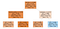New Decision Tree

# Method Three: Random Forest

## 1. Fit, Predict, and Accuracy Score:

`from sklearn.ensemble import RandomForestClassifierrf_model = RandomForestClassifier(random_state = 2020)rf_model.fit(X_train,y_train)`
`y_pred_rf = rf_model.predict(X_test)acc_rf = accuracy_score(y_test,y_pred_rf)print('Accuracy = ', acc_rf)`
`Accuracy =  0.9166666666666666`

## 2. Overfitting:

`scores = cross_val_score(rf_model, X, y, cv=5)print("Cross Validation Score: ",scores.mean())`
`Cross Validation Score:  0.8680466300940439`
`y_pred_train = rf_model.predict(X_train)print(metrics.accuracy_score(y_train, y_pred_train))`
`Training Accuracy = 1.0`

## 3. Tune the Model’s Parameters:

`print(rf_model.get_params())`
`{'bootstrap': True, 'ccp_alpha': 0.0, 'class_weight': None, 'criterion': 'gini', 'max_depth': None, 'max_features': 'auto', 'max_leaf_nodes': None, 'max_samples': None, 'min_impurity_decrease': 0.0, 'min_impurity_split': None, 'min_samples_leaf': 1, 'min_samples_split': 2, 'min_weight_fraction_leaf': 0.0, 'n_estimators': 100, 'n_jobs': None, 'oob_score': False, 'random_state': 2020, 'verbose': 0, 'warm_start': False}`
`random_grid = {'max_depth': [1, 5, 10, 15],'n_estimators': [100,200,300,400,500,600]}rf_random = RandomizedSearchCV(rf_model,random_grid, n_iter = 50, cv = 5,random_state = 2020)rf_random.fit(X_train, y_train)print(rf_random.best_params_)`
`{'n_estimators': 300, 'max_depth': 10}`
`param_dist = {"max_depth":[7,8,9,10,11,12,13,14,15],      "n_estimators":[280,300,320,350,380,400,420,450,480]}rf_cv = GridSearchCV(rf_model, param_dist, cv=5)rf_cv.fit(X_train,y_train)print(rf_cv.best_params_)`
`{'max_depth': 14, 'n_estimators': 450}`
`rf_new = RandomForestClassifier(n_estimators = 450, max_depth =  14, random_state = 2020)rf_new.fit(X_train,y_train)y_pred_rf = rf_new.predict(X_test)acc_rf = accuracy_score(y_test,y_pred_rf)print('Accuracy = ', acc_rf)scores = cross_val_score(rf_new, X, y, cv=5)print("Cross Validation Score: ",scores.mean())`
`New Random Forest Accuracy = 0.9166666666666666New Cross Validation Score =  0.868669670846395  `

# Feature Importance:

`imp_rf = pd.DataFrame(zip(X_train.columns, rf_model.feature_importances_),columns = ["feature", "importance"])imp_rf.set_index("feature", inplace=True)imp_rf.sort_values(by = "importance", ascending = False, inplace = True)imp_rf.head()`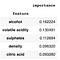Feature Importance
`imp_rf.plot.barh(figsize=(10,10))plt.show()`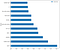Feature Importance Graph

# Conclusion:

`import numpy as npprint(np.average(df[df["good wine"] == "yes"].alcohol))print(np.average(df[df["good wine"] == "no"].alcohol))`
`Good Wine =  11.518049155145931 Regular Wine =  10.251037144235408`
`print(np.average(df[df["good wine"] == "yes"].sulphates))print(np.average(df[df["good wine"] == "no"].sulphates))`
`Good Wine =  0.7434562211981566 Regular Wine =  0.6447539797395079`
`df_good = df[df["good wine"] == "yes"]df_bad = df[df["good wine"] == "no"]print(np.average(df_good["volatile acidity"]))print(np.average(df_bad["volatile acidity"]))`
`Good Wine = 0.4055299539170507 Regular Wine = 0.5470224312590448`

USC Sophomore Majoring in CSBA

## More from Jingyi Fang

USC Sophomore Majoring in CSBA